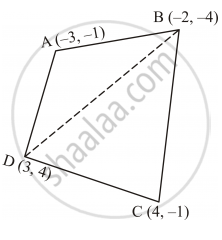Share

# Find the Area of the Quadrilateral Abcd, Whose Vertices Are A(−3, −1), B (−2, −4), C(4, − 1) and D (3, 4). - CBSE Class 10 - Mathematics

ConceptCoordinate Geometry Examples and Solutions

#### Question

Find the area of the quadrilateral ABCD, whose vertices are A(−3, −1), B (−2, −4), C(4, − 1) and D (3, 4).

#### Solution

The given quadrilateral i.e., ABCD whose vertices are A (−3, −1), B (−2, −4), C (4, −1) and D (3, 4) can be drawn as follows:Here, B is joined with D.

We know that the area of a triangle whose vertices are (x1 , y1 ), (x2 , y2 ) and (x3 , y3 ) is given by

=1/2[x_1(y_2-y_3)+x_2(y_3-y_1)+x_3(y_1-y_2)]

=1/2[-3(-8)-2(5)+3(3)]

=1/2[24-10+9]

=23/2

=11.5 sq.inits

∴ar(ΔABD)

=1/2[-3(-4-4)+(-2)(4+1)+3(-1+4)]

∴ar (ΔCDB)

=1/2[4(4+4)+3(-4+1)+(-2)(-1-4)]

=1/2[(4xx8)+(3x-3)-2xx(-5)]

=1/2[32-9+10]

=33/2

=16.5 sp.unit

Thus, ar (ABCD) = ar (ΔABD) + ar (ΔCDB) = (11.5 + 16.5) sq units = 28 sq units

Is there an error in this question or solution?

#### Video TutorialsVIEW ALL 

Solution Find the Area of the Quadrilateral Abcd, Whose Vertices Are A(−3, −1), B (−2, −4), C(4, − 1) and D (3, 4). Concept: Coordinate Geometry Examples and Solutions.
S